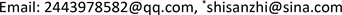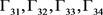﻿ 基于结构方程模型的大学生时间管理对学业影响的相关性分析 Analysis about Influence of College Students’ Time Management on Academic Based on Structural Equation Modeling

Statistics and Application
Vol.07 No.01(2018), Article ID:23700,7 pages
10.12677/SA.2018.71004

Analysis about Influence of College Students’ Time Management on Academic Based on Structural Equation Modeling

Wenwen Li, Jiawei Fan, Yuchao He, Jianyong Xue, Yahui Dong, Sanzhi Shi*

College of Science, Changchun University of Science and Technology, Changchun JilinReceived: Jan. 17th, 2018; accepted: Jan. 31st, 2018; published: Feb. 7th, 2018ABSTRACT

The structural equation model is a mathematical analysis method to establish and statistically predict the causality of multivariate variables. From the perspective of psychology, this article sets the academic as the four dimensions of learning ability, creative thinking, verbal expression ability and communication and cooperation ability, setting time as the three dimensions of time value, time monitoring and time efficacy. 700 questionnaires were distributed to students in four colleges and universities in Changchun, and the questionnaire reliability was analyzed by using SPSS statistical software. The reliability of the questionnaire was 0.893, indicating good reliability. Using the AMOS software to establish the model coefficient estimation statistic and the structural equation model path map, the P values of all the path coefficients are all below the significance level of 0.05, indicating that there is a significant positive correlation between the time management and the academic level of undergraduates and the related structural equations are established. The results show that good time management can not only improve students' learning performance, but also lay a good foundation for further socialization of personality.

Keywords:Structural Equation Model, Correlation Analysis, Time Management, College Students, Academic1. 引言

2. 结构方程模型原理和模型建构

1) 测量方程

$X={\Lambda }_{x}\xi +\delta ,\text{\hspace{0.17em}}\text{\hspace{0.17em}}Y={\Lambda }_{y}\eta +e$

2) 结构方程

$\eta =B\eta +\Gamma \xi +\zeta$

3. 时间管理与学业相关性的建模

3.1. 变量选取

$Y$ 是由内生指标组成的向量，分量对应文中的 ${Q}_{1}~{Q}_{11}$$X$ 是由外生指标组成的向量，分量分别对应本文中的 ${Q}_{12}~{Q}_{20}$

$e$ 表示外生指标X的误差项和内生指标Y的误差项。在本文中内生指标 $Y$ 的误差项为 ${e}_{1}~{e}_{11}$ 外生指标 $X$ 的误差项为 ${e}_{12}~{e}_{20}$$\zeta$ 表示结构方程的残差项，本文中 ${\zeta }_{1}$ 为学习能力的残差项， ${\zeta }_{2}$ 为创造性思维的残差项， ${\zeta }_{3}$ 为语言表达能力的残差项， ${\zeta }_{4}$ 为交流合作能力的残差项； $\lambda$ 为外生指标与外生潜变量之间的关系以及内生指标与内生潜变量之间的关系， ${Q}_{1},{Q}_{2},{Q}_{3}$ 对学习能力的关系记为 ${\lambda }_{1},{\lambda }_{2},{\lambda }_{3}$${Q}_{4},{Q}_{5},{Q}_{6}$ 和创造性思维的关系记为 ${\lambda }_{4},{\lambda }_{5},{\lambda }_{6}$${Q}_{7},{Q}_{8}$ 和语言表达能力的关系记为 ${\lambda }_{7},{\lambda }_{8}$${Q}_{9},{Q}_{10},{Q}_{11}$ 和交流合作能力之间的关系记为 ${\lambda }_{9},{\lambda }_{10},{\lambda }_{11}$${Q}_{12},{Q}_{13}$ 和时间价值感之间的关系记为 ${\lambda }_{12},{\lambda }_{13}$${Q}_{14},{Q}_{15},{Q}_{16},{Q}_{17}$ 和时间监控感之间的关系记为 ${\lambda }_{14},{\lambda }_{15},{\lambda }_{16},{\lambda }_{17}$${Q}_{18},{Q}_{19},{Q}_{20}$ 对时间效能感之间的关系记为 ${\lambda }_{18},{\lambda }_{19},{\lambda }_{20}$

$\Gamma$ 是外生潜变量对内生潜变量的影响，在本文中时间价值感对学习能力，创造性思维，语言表达能力，交流合作能力的关系记为 ${\Gamma }_{11},{\Gamma }_{12},{\Gamma }_{13},{\Gamma }_{14}$ ，时间控制感学习能力，创造性思维，语言表达能力，交流合作能力的关系记为 ${\Gamma }_{21},{\Gamma }_{22},{\Gamma }_{23},{\Gamma }_{24}$ ，时间效能感对学习能力，创造性思维，语言表达能力，交流合作能力的关系记为 ${\Gamma }_{31},{\Gamma }_{32},{\Gamma }_{33},{\Gamma }_{34}$

3.2. AMOS分析

***在0.05水平(双侧)上显著相关。Figure 1. Structural equation model path map

3.3. 建立结构方程模型

${Y}_{i}={\eta }_{1}{\lambda }_{i}+{e}_{i}\left(i=1,2,3;{\lambda }_{i}=0.50,0.66,0.52;{e}_{i}=0.25,0.43,0,27\right)$

${Y}_{i}={\eta }_{2}{\lambda }_{i}+{e}_{i}\left(i=4,5,6;{\lambda }_{i}=0.54,0.71,0.60;{e}_{i}=0.29,0.50,0.36\right)$

${Y}_{i}={\eta }_{3}{\lambda }_{i}+{e}_{i}\left(i=7,8;{\lambda }_{i}=0.70,0.76;{e}_{i}=0.49,0.58\right)$

${Y}_{i}={\eta }_{4}{\lambda }_{i}+{e}_{i}\left(i=9,10,11;{\lambda }_{i}=0.75,0.74,0.68;{e}_{i}=0.56,0.55,0.45\right)$

${X}_{i}={\xi }_{1}{\lambda }_{i}+{e}_{i}\left(i=12,13;{\lambda }_{i}=0.14,0.53;{e}_{i}=0.28,0.35\right)$

${X}_{i}={\xi }_{2}{\lambda }_{i}+{e}_{i}\left(i=14,15,16,17;{\lambda }_{i}=0.59,0.75,0.71,0.71;{e}_{i}=0.35,0.57,0.51,0.51\right)$

${X}_{i}={\xi }_{3}{\lambda }_{i}+{e}_{i}\left(i=18,19,20;{\lambda }_{i}=0.23,0.53,0.43;{e}_{i}=0.05,0.28,0.19\right)$

${\Gamma }_{11},{\Gamma }_{13},{\Gamma }_{14},{\Gamma }_{22},{\Gamma }_{24},{\Gamma }_{31},{\Gamma }_{32},{\Gamma }_{33}$ 由于其所表示关系的项之间的相关性较小，以及用SEM模型验证路径系数的P值不在0.05显著性水平下，把值设为0，则可得：

$\left(\begin{array}{c}{\eta }_{1}\\ {\eta }_{2}\\ {\eta }_{3}\\ {\eta }_{4}\end{array}\right)=\left(\begin{array}{ccc}0& 0.78& 0\\ 0.93& 0& 0\\ 0& 0.87& 0\\ 0& 0& 0.93\end{array}\right)\left(\begin{array}{c}{\xi }_{1}\\ {\xi }_{2}\\ {\xi }_{3}\end{array}\right)+\left(\begin{array}{c}0.80\\ 0.86\\ 0.75\\ 0.88\end{array}\right)$

4. 模型结果分析

4.1. 问卷可信度分析

4.2. 学生时间管理和学习能力的相关性总体分析

4.3. 从不同的维度分析

1) 心理学研究表明，女性多属于场依存型，男性则多属于场独立型。步入大学，学生可以自由支配的时间增多，师长的约束减少，男生的独立适应能力明显高于女生，导致男生的时间管理倾向总体要强于女生。但女生因为从小被灌以做事认真、踏实、稳重的思想，所以在时间监控感上要优于男生，更容易在规定时间内完成学习任务。学习能力、交流合作能力要优于男生。同时有研究指出，男生和女生的大脑发育从小就是不一样的，这就导致女生的抽象思维和逻辑思维不如男生，所以男生的创造性思维要优于女生，当然语言表达能力也要优于女生。

2) 从不同地区的角度来说，南方大都是经济发达省份，人口较多，竞争也就相对比较激烈，这就使得南方的教学质量高于北方的教学质量，学生的学习观念比较强，使得其拥有较强的学习能力。相比之下，北方学生没有了地域优势，只能靠自身的努力来发展，所以北方学生对自身的要求严格，在时间管理上要优于南方学生。

3) 对于不同高校的学生，985和211高校的学生学习能力及时间管理能力相比一本学生和二本学生Table 3. Student time management and learning ability of the overall analysis of the correlation table

4) 从心理学角度来说，初到一个陌生的环境，人本能的想要证明自己，这在211,985名校中尤为明显。但与此同时，大一的学生并没有完全掌握高中与大学学习方法上的不同，导致学习效果并不明显，相比之下，大二、大三的学生学习能力较强，同时，随着学科竞赛的加入，高年级学生的交流合作及语言表达能力也得到了加强。

5. 结论

Analysis about Influence of College Students’ Time Management on Academic Based on Structural Equation Modeling[J]. 统计学与应用, 2018, 07(01): 25-31. http://dx.doi.org/10.12677/SA.2018.71004

1. 1. 黄希庭, 张志杰. 青少年时间管理倾向量表的编制[J]. 心理学报, 2001, 33(4): 338-343.

2. 2. 魏佳. 大学生时间管理倾向与学业成绩的相关分析[J]. 高等教育, 2015(35): 34-40.

3. 3. 李茹锦. 不同成就目标定向大学生的时间管理倾向及其与学业成绩的关系[D]: [硕士学位论文]. 济南: 山东师范大学, 2008: 168-194.

4. 4. 侯杰泰, 温忠麟, 成子娟. 结构方程模型及其应用[M]. 北京: 教育科学出版社, 2014.

5. 5. Janssens, H., Braeckman, L., De Clercq, B., Casini, A., De Bacquer, D., Kittel, F. and Clays. E. (2016) The Indirect Association of Job Strain with Long-Term Sickness Absence Through Bullying: A Mediation Analysis Using Structural Equation Modeling. BMC Public Health, 6, 851-863. https://doi.org/10.1186/s12889-016-3522-y

6. 6. Fulk, F., Succop, P., Hilbert, T.J., Beidler, C., Brown, D., Reponen, T. and Haynes, E.N. (2016) Pathways of Inhalation Exposure to Manganese in Children Living near a Ferromanganese Reﬁnery: A Structural Equation Modeling Approach. Science of the Total Environment, 21, 332-340.

7. 7. Lee, S. and Ahn, H. (2009) Structural Equation Model for EDI Controls: Controls Design Per-spective. Expert Systems with Applications, 36, 1731-1749. https://doi.org/10.1016/j.eswa.2007.12.008

8. 8. 郭志刚. 社会统计分析方法——SPSS软件应用[M]. 北京: 中国人民大学出版社, 1999.